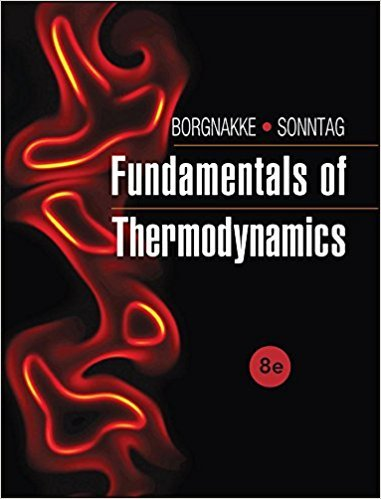×
Get Full Access to Fundamentals Of Thermodynamcs - 8 Edition - Chapter 3 - Problem 36hp
Get Full Access to Fundamentals Of Thermodynamcs - 8 Edition - Chapter 3 - Problem 36hp

×

# A steel ball weighing 5 kg rolls horizontally at a rate ofISBN: 9781118131992 58

## Solution for problem 36HP Chapter 3

Fundamentals of Thermodynamcs | 8th Edition

• Textbook Solutions
• 2901 Step-by-step solutions solved by professors and subject experts
• Get 24/7 help from StudySoup virtual teaching assistantsFundamentals of Thermodynamcs | 8th Edition

4 5 1 409 Reviews
11
3
Problem 36HP

Problem 36HP

A steel ball weighing 5 kg rolls horizontally at a rate of 10 m/s. If it rolls up an incline, how high up will it be when it comes to rest, assuming standard gravitation?

Step-by-Step Solution:

Solution 36HP

Step 1 of 2

Here we have to calculate how high upwards on a inclined plane a ball can reach, if the same ball weighing 5 kg rolls at a speed of 10 m/s.

Given data,

Mass of ball,Speed,Acceleration due to gravity,To find,

Height,Step 2 of 2

##### ISBN: 9781118131992

Unlock Textbook Solution